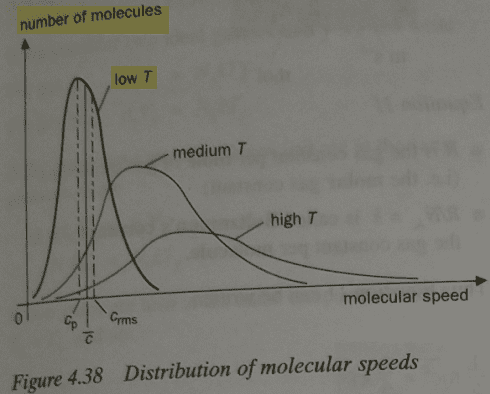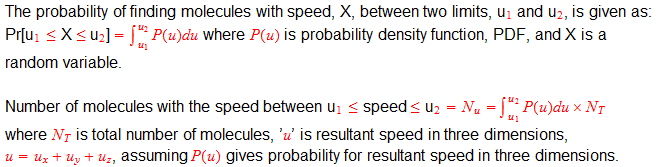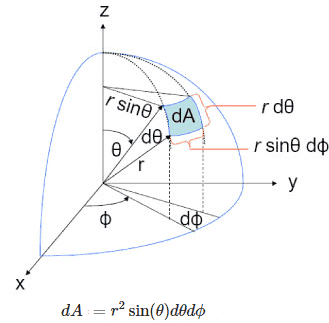# Boltzmann distribution and the number of molecules with a certain velocity

PainterGuy
Hi,

You could skip these details and find the main question at the bottom. I added the details for the sake completeness and context. Thanks.

Boltzmann distribution of molecular speeds provides an insight into the different speeds the molecules of a gas are moving around with. It provides you with the probability of finding the fraction of molecules moving around with in a certain speed range.

Sometimes a graph as the histogram of data is used for the entire population of molecules and then theoretical curve is used to approximate this data. Since, the molecules are undergoing changing velocity all the time so we can pretend that the distribution is a snapshot taken at one instant of time

The following links illustrate the point.
1: https://en.wikipedia.org/wiki/File:Simulation_of_gas_for_relaxation_demonstration.gif
2: https://chem.libretexts.org/Bookshelves/General_Chemistry/Book:_ChemPRIME_(Moore_et_al.)/09Gases/9.16:_Kinetic_Theory_of_Gases:_The_Distribution_of_Molecular_Speeds
3: https://encrypted-tbn0.gstatic.com/...1eNIvw6VcVdyO5lIGVaOc0EFawkji88A5Jg_Ych4Y0Q90

We can see below that population distribution curve is asymmetric - negatively skewed; it's not normal curve. I believe that they should have used a label "probability" instead of "number of molecules". I'm focusing on the curve with label "low T". Cp is the most probable speed, i.e. modal speed, c_bar is mean speed, c_rms is root mean square speed.Mathematically, it can be written."In a more precise sense, the PDF is used to specify the probability of the random variable falling within a particular range of values, as opposed to taking on anyone value. This probability is given by the integral of this variable's PDF over that range—that is, it is given by the area under the density function but above the horizontal axis and between the lowest and greatest values of the range. The probability density function is nonnegative everywhere, and its integral over the entire space is equal to one."
Source: https://en.wikipedia.org/wiki/Probability_density_function

A PDF gives area under the curve so just like when you are doing integration you need two limits to find the area and using one value doesn't mean anything.

Watch this video from 4:42 onward to know more about PDF; remove the parentheses:
https://www.youtu(be.com/watch?v=mtH1fmUVkfE)

The following link explains Boltzmann distribution:

You can find the actual probability density function used here, https://imagizer.imageshack.com/img924/8804/GxnuI2.jpg. It only gives you the probability of speed in one dimensions. The speed in three dimensions is given here, https://imagizer.imageshack.com/img921/2119/BzLs8Q.jpg. This is the source for both images: https://www.tec-science.com/thermod...-the-maxwell-boltzmann-distribution-function/ . Also check this link: https://www.tec-science.com/thermodynamics/kinetic-theory-of-gases/maxwell-boltzmann-distribution/

Question:
Now I'm coming to my question. The provided provided by Maxwell–Boltzmann distribution deals with speed and not velocity. How could I find the number of molecules in a gas traveling in a certain direction with speed between u and u+du where du is an infinitesimal increase in the speed? The speed plus direction is velocity. Thanks for the help.

Note to self:
check related question with picture of molecular speeds: https://www.physicsforums.com/threa...on-discrete-to-continuous.976040/post-6223236
check note to self:
https://www.physicsforums.com/threa...on-discrete-to-continuous.976040/post-6221193https://chem.libretexts.org/Bookshelves/Physical_and_Theoretical_Chemistry_Textbook_Maps/Map:_Physical_Chemistry_(McQuarrie_and_Simon)/27:_The_Kinetic_Theory_of_Gases/27.3:_The_Distribution_of_Molecular_Speeds_is_Given_by_the_Maxwell-Boltzmann_Distribution
https://chem.libretexts.org/Bookshe...ing_Gas_Properties_to_Kinetic_Theory_of_Gases
https://en.wikipedia.org/wiki/Maxwell–Boltzmann_distributionsolid angle; remove the parentheses: https://www.yout(ube.com/watch?v=RMJucQJ1NGo)
spherical coordinates; remove the parentheses: https://www.youtu(be.com/watch?v=FDyenWWlPdU)
https://hsm.stackexchange.com/quest...ion-of-velocities-in-a-gas-during-an-exam-set

Homework Helper
Gold Member
To be able to answer questions about the distribution of direction of velocity , I believe we must have information about the distribution of each individual component ##v_x,v_y,v_z## in cartesian coordinates (or ##v_{\rho},v_{\theta},v_{\phi}## in spherical coordinates).

Are you given the velocity distributions for each individual component?

•PainterGuy
PainterGuy
To be able to answer questions about the distribution of direction of velocity , I believe we must have information about the distribution of each individual component ##v_x,v_y,v_z## in cartesian coordinates (or ##v_{\rho},v_{\theta},v_{\phi}## in spherical coordinates).

Are you given the velocity distributions for each individual component?

No. I don't have any more information. I was just thinking about this problem and thought that I should try to figure it out that how such a problem could be solved. Thanks.

Last edited:
mpresic3
Usually, the MB distribution is isotropic meaning (colloquially) one direction is as good as another. The motion is equally likely to be in any direction. One well-known exception to this is an isothermal atmosphere under constant gravity. The particles are more likely to be near the surface of the planet than higher up. Otherwise the motion in x-y (parallel to the planet) will be equally likely.

•PainterGuy
PainterGuy
Usually, the MB distribution is isotropic meaning (colloquially) one direction is as good as another. The motion is equally likely to be in any direction.

Thank you!

I don't follow your point because there should be a way to know the number or probability of molecules moving in a particular direction. For example, only a few would be moving exactly in x-axis direction; likewise some would be moving in z-axis direction.

I believe we must have information about the distribution of each individual component ##v_x,v_y,v_z## in cartesian coordinates (or ##v_{\rho},v_{\theta},v_{\phi}## in spherical coordinates).

We can assume some values values for ##v_x,v_y,v_z## in cartesian coordinates.

mpresic3
The probability that any particle would be traveling "exactly" along the x (or any other) precisely defined direction is zero. The gaussian distribution and MB distributions are continuous distributions.

If you are acquainted with the differential area element (e.g. in spherical coordinates, this is how you would do it.

The differential area element in spherical coordinates is dA = sin(theta) d(theta) d(phi). Phi runs from 0 to 2 * pi, and theta runs from 0 to pi.

Perform the double integral with these limits gives you a total area of 4 * pi.

Now suppose you want to determine the number of particles within 5 degrees of the z-axis. so that theta is now limited to between zero and pi / 36 (= 5 degrees)

You would find the total area as ( (2*pi) times integral from 0 to pi/36 of sin(theta) d(theta) )
= 2 pi (1-cos(pi/36))

Because as I suggested one direction is as good as another, the probability of the particle within 5 degrees of the z axis is the ratio of this value over the total probability (area) namely 4 pi.

So the probability of the angle being within 5 degrees of z is 2 * pi * (1 - cos(pi/36)) / 4 * pi =

= 1/2 * (1 - cos(pi/36)). You can generalize this analysis to other angles similarly

•PainterGuy and Delta2
Homework Helper
Gold Member
I find @mpresic3 analysis(his post needs abit of "refinement" though and needs Latex to be written properly) mostly correct (given the assumption that all directions have equal probability which certainly holds if the shape of the container of the gas is spherical but I am not 100% sure for other shapes) and it is straightforward to generalize it for direction within any range of angles ##\Delta\phi## and ##\Delta\theta##, where ##\phi## is the azimuth angle and ##\theta## the elevation angle (spherical coordinates).
I just want to say that if we are interested not only for a range of directions but for a range of speeds(the magnitude of velocity) as well we just multiply that probability by the probability ##P(v_0-\Delta v<v<v_0+\Delta v)## we find by integrating Maxwell's-Boltzman distribution within the given range of speeds.

•PainterGuy
PainterGuy
You would find the total area as ( (2*pi) times integral from 0 to pi/36 of sin(theta) d(theta) )
= 2 pi (1-cos(pi/36))

I'm not able to understand what is being done in the quoted step? I understand that in your calculation you have assumed r=1 but what is "sin(theta) d(theta)"? It's not an area element. Could you please elaborate a bit? Thank you.mpresic3
The volume element is r squared sin theta dr dtheta d phi. The area element (fix r at 1), is sine (theta) d theta d phi. Integrate over phi first because we expect the number is not dependent on azimuth angle. This gives you an area element(after the integration over phi) as 2 * pi * sine (theta) d theta.

to get the number within a cone of angle theta/36 (say) you now integrate 2 * pi * sine(theta) d theta from 0 to pi / 36. If you divide this amount by 4*pi (the total area of the sphere), you get the fraction of area of the "cone" within the 5 degrees of the pole, to the total area of the sphere. Because the particles are just as likely to be moving in any direction, the number within 5 degrees of the pole will be the total number times this fraction.

•PainterGuy
PainterGuy

If you don't mind I'd like to return to this thread after a few days. Had to take care of few other things. Thank you for the understanding.